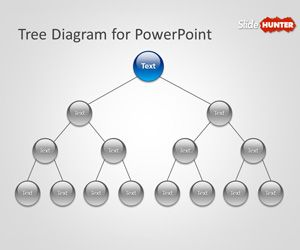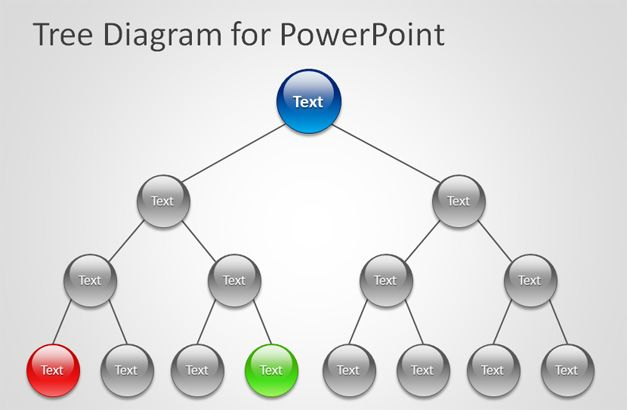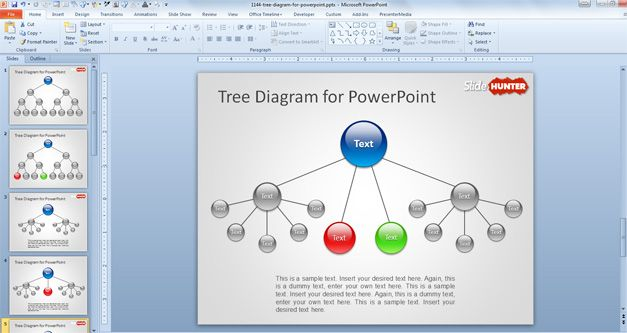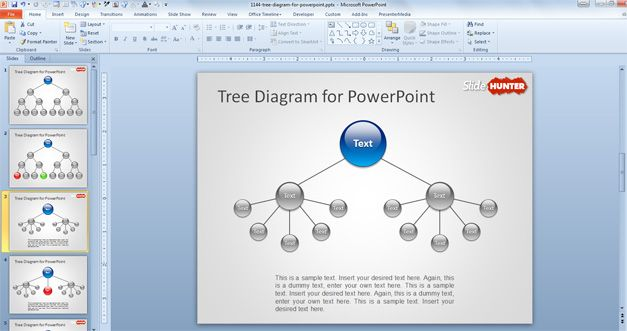Home   Free PowerPoint Templates   Design   Tree Diagram for PowerPoint

## Tree Diagram for PowerPointFree Tree Diagram for PowerPoint is a simple but effective PowerPoint template containing a blank tree diagram that you can customize for your own needs. This free diagram template for PowerPoint presentations helps you to make organizational charts in PowerPoint as well as family trees. You can virtually create any hierarchical representation using PowerPoint and this tree diagram.

There are many different types of tree diagrams but they have some common properties. You can use tree diagrams in many different sectors, applications and for different purposes. Tree diagrams help to visualize hierarchical data  and it is common to see horizontal tree diagrams where the root node is on the top and then the branches below. However, some other tree diagrams may be represented with the root at the bottom of the layout. The family trees are a case of the latter.A tree diagram is simply a way of representing a sequence of events using a tree structure, it helps to represent the hierarchical nature of a structure in a graphical form. Tree diagrams are particularly useful in Probability Theory but also in other fields of interest and studies, for example for decision trees, game tree, parse tree in linguistics to visually represent the structure of a sentence, syllable or phonological feature geometry, in biology to represent phylogenetics or in physics to represent a Feynman diagram.

Another kind of tree diagrams are the probability tree diagrams, widely used for statistics and maths. Probability tree diagrams may have rich information added to the edges of the tree (the probability). You can learn more about tree diagrams used in Maths here or an introductory to tree diagrams.

This free tree diagram PowerPoint template is just a simple but effective PowerPoint template with tree diagrams example that you can use as a blank template to prepare your presentations with rich tree diagrams. You can use this free template to create awesome tree diagrams using PowerPoint shapes. The template contains many different tree layouts and PowerPoint slides with tree diagram styles. For example, you can download and use the simple tree diagram slide design with a root on top of the layout and branches, or you can use the 3rd. slide to make attractive tree diagrams with nodes in different sizes.If your goal is to make an impressive PowerPoint presentation to help visualize the data then using this tree diagram you can help to achieve this goal. The audience can see the whole of a organization structure using a tree diagram and you can empathize the important nodes by changing the circle sizes. Also, you can use different colors as a legend to represent different kind of information.In this latest figure we can see another example of tree diagram layout where there are two main branches below the top root node and then each branch is diversified in other branches and nodes.

There are many other visualization tools that you can use to make stunning presentations in PowerPoint, for example the Cluster diagrams for PowerPoint can help to visualize a cluster or make cluster analysis using Microsoft PowerPoint.

License: cc by 2.0